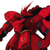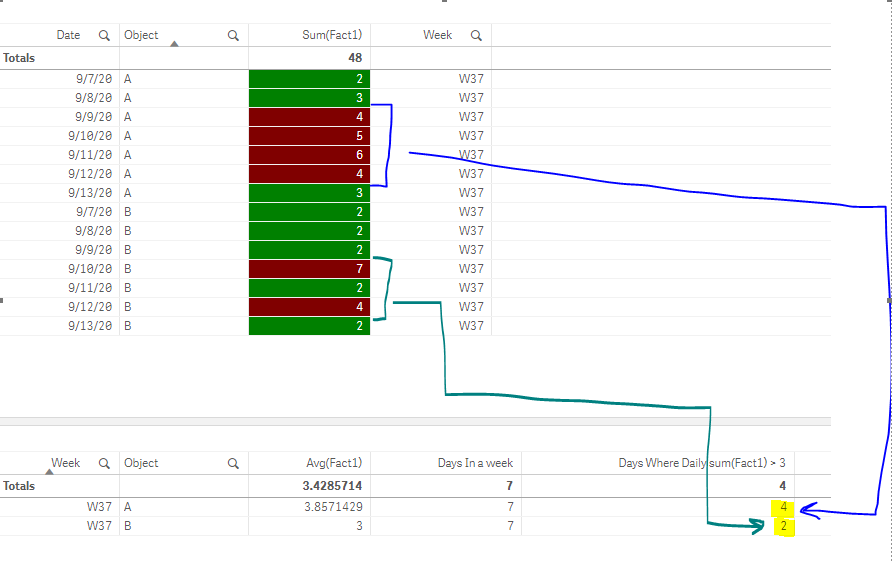# App Development

Announcements
Qlik Insider: SaaS capabilities for Data Integration and Analytics, May 25th! Register Now
cancel
Showing results for
Did you mean:Creator

## Calculate for N number of objects per week, how many days a KPI’s daily value crossed a threshold.

Hey Qliksters,

I have a use-case where a user wants to calculate for N number of objects per week, how many days a KPI’s daily value crossed a threshold.

As an example:

Given these rows below, in a separate table, calculate per object per week how many days had a value greater than 3.Some conditions/things to keep in mind:

1. The actual data is at the 15 minute level, so I don’t think we can just write: count({<Fact1 = {">3"}>}distinct Date) because the threshold is based on the daily value)
2. There are 800 million+ fact rows in the actual data set, so it would not be ideal to have a unique key per row of data to "force" the  set analysis to calculate row-by-row. I tried and hit a calculation timeout
• count({<uniqueRowKey= {"= sum(aggr(sum(Fact1),uniqueRowKey)) > 3"}>}distinct Date)

Any tips and tricks?

Thanks!!!

S

0 Replies